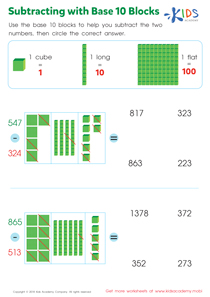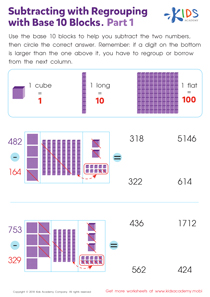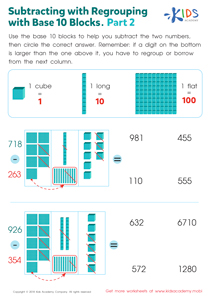# Math Lesson - Place Value to Subtract, Grade 3

• ### Activity 1 / Subtracting with Base 10 Blocks WorksheetIs your child struggling with subtracting larger 3-digit numbers? If so, he or she isn’t alone! Even before learning to regroup, subtraction is tough, and kids often need a visual way to understand the concept. This PDF worksheet from Kids Academy uses colorful green base blocks to show your children the idea behind subtraction. Use the visuals to help your little learner subtract tough 3-digit numbers. Simply subtract each number beginning with the tens column, and use the images for assistance and clarification. Then circle the correct answer to complete this helpful math sheet!

• ### Activity 2 / Subtracting with Regrouping with Base 10 Blocks. Part1 WorksheetSubtracting with regrouping is a tough concept to master, but a visual aid can go a long way in helping little learners to get it down! This helpful worksheet features colorful base ten blocks that show kids exactly how to regroup by borrowing from the next column. Walk your child through each problem on the page subtracting each column starting from the ones. When it’s time to regroup, review the concept with kids, and look at the picture to help them understand. Complete the sheet by circling the correct answer.

• ### Activity 3 / Subtracting with Regrouping with Base 10 Blocks. Part 2 WorksheetLearning to subtract with regrouping takes a lot of practice! Give your kids what they need to be successful and use this handy printable worksheet from Kids Academy! Your child will be tasked with completing two 3-digit subtraction problems, both requiring kids to regroup, borrowing from the next column. Colorful base blocks are used to foster a deeper understanding of regrouping, showing students the concept in an easy-to-understand way!

?